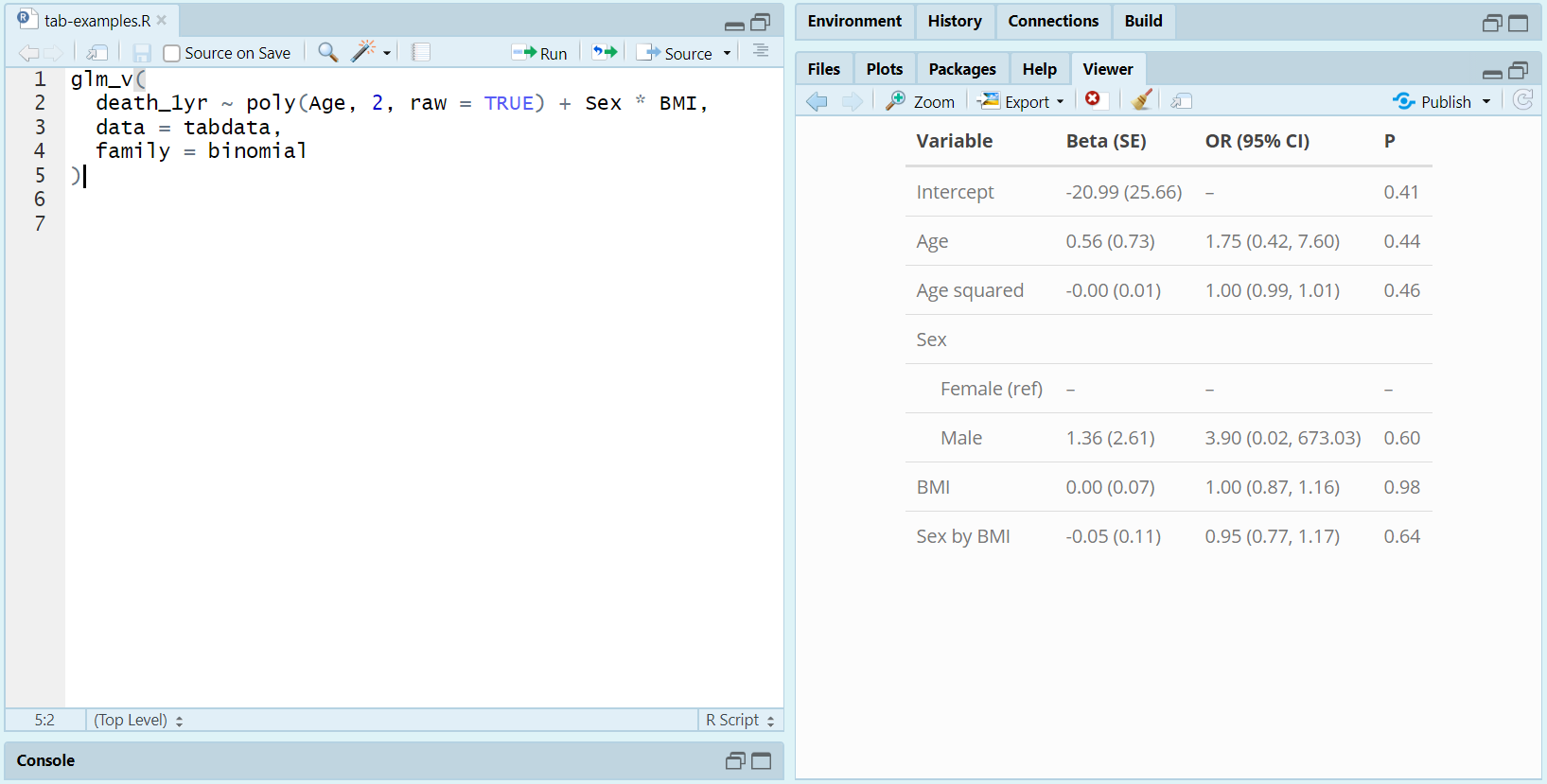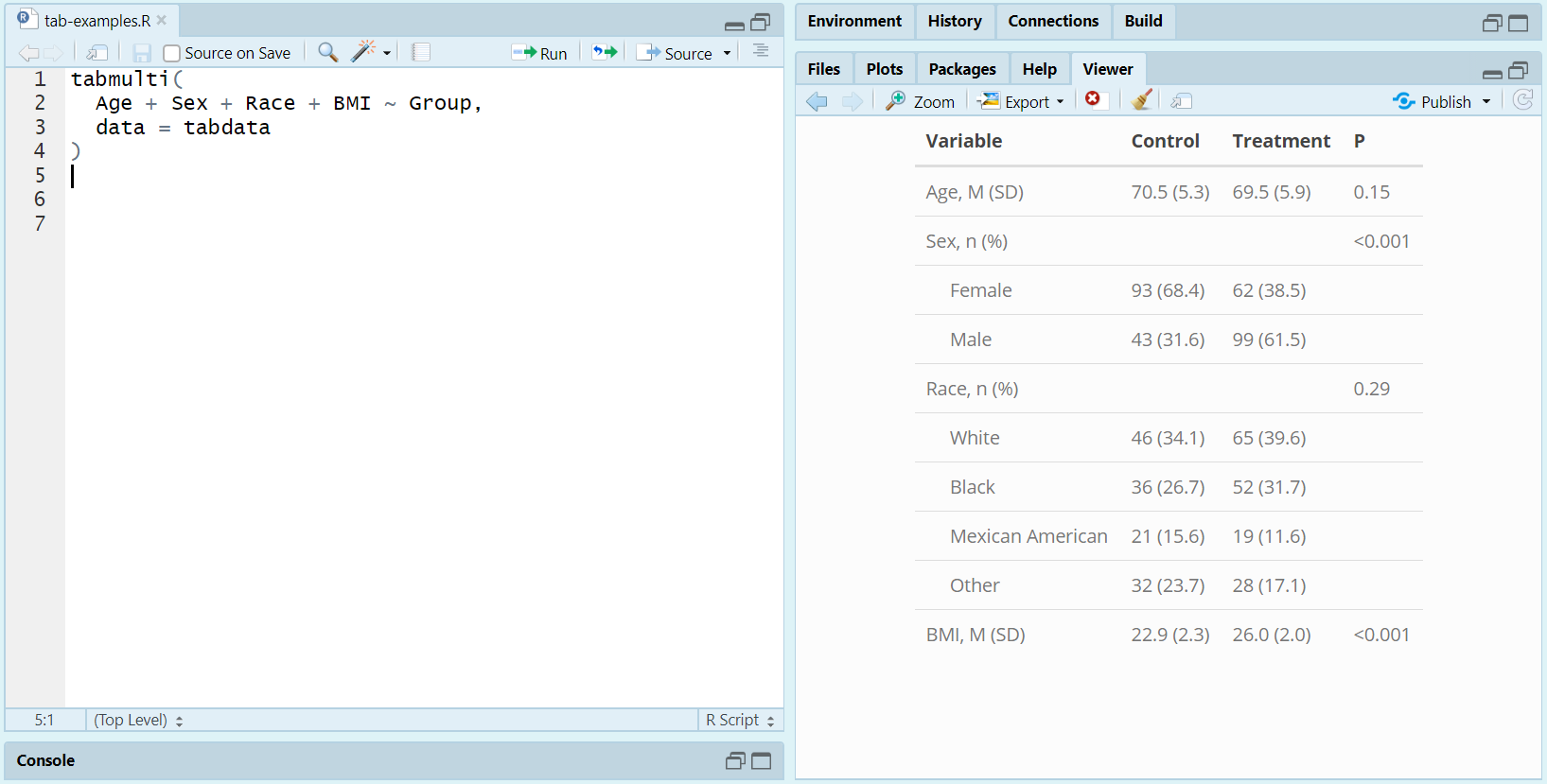# Summary Tables with ‘tab’

## Installation

You can install and load tab from GitHub via the following code:

devtools::install_github("vandomed/tab")
library("tab")

## Functions

The main purpose of tab is to create neatly formatted summary tables for papers and presentations. The following functions are included:

• glm_v prints a GLM summary table to the RStudio Viewer
• tabglm summarizes generalized linear models (GLM’s) fit via glm or survey::svyglm
• tabgee summarizes generalized estimating equation models (GEE’s) fit via gee::gee
• tabcoxph summarizes Cox Proportional Hazards models fit via survival::coxph or survey::svycoxph
• tabmulti compares variables across two or more groups, e.g. to create a “Table 1”
• tabmulti.svy does the same thing as tabmulti but for complex survey data

## Regression summaries with just 2 extra keystrokes

To summarize a fitted generalized linear model, simply call glm_v as you would glm. The result will be a formatted summary table printed to the RStudio Viewer. Here’s an example for logistic regression:

glm_v(
death_1yr ~ poly(Age, 2, raw = TRUE) + Sex * BMI,
data = tabdata,
family = binomial
)From here, you can “snip” the summary table and save it as a figure (as I did for this README) or copy directly from the Viewer and paste outside of R.

For more flexibility, see tabglm. That function lets you control things like what columns to present, how categorical predictors are presented, and so on.

## Summary tables for continuous and categorical variables

You can use tabmulti to summarize variables across two or more groups, using a formula interface. Here’s an example:

tabmulti(Age + Sex + Race + BMI ~ Group, data = tabdata)## Compatibility with Markdown/Knitr

The functions all return kable objects, so they should work perfectly well in R Markdown and knitr documents.